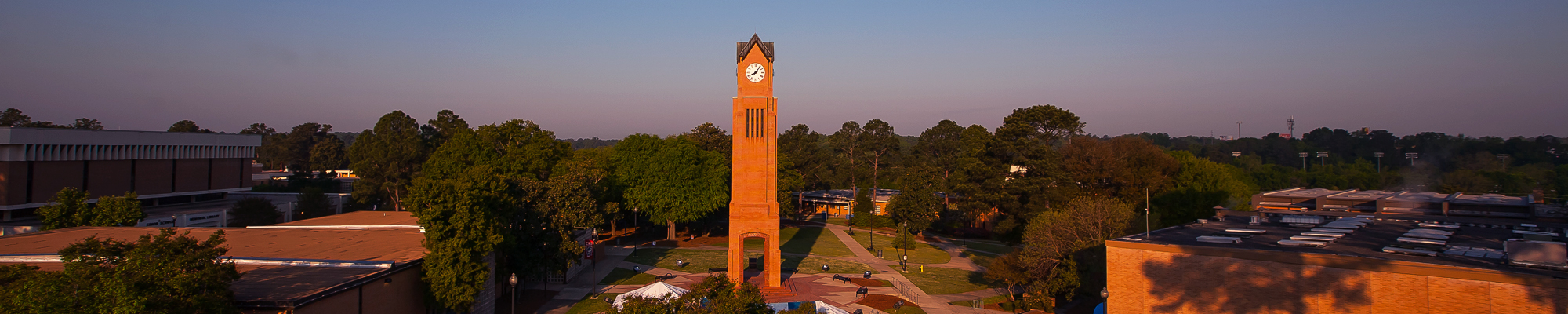## Compare Courses

MATH 1113. Pre-Calculus (4-0-4) Prerequisite: MATH 1111 with a grade of "C" or better or an appropriate math placement score. This course is designed to prepare students for calculus, physics, and related technical subjects. Topics include algebraic and transcendental functions and an intensive study of trigonometric functions.

MATH 3154. Introduction to Mathematical Proofs I (3-0-3) Prerequisite: MATH 1131 with a minimum grade of "C". Preparation in mathematical reasoning and proof-writing necessary for upper division course work in mathematics. Topics include fundamentals of logic, techniques of proof, sets and relations, equivalence relations and partitions, and mathematical induction.

MATH 3155. Introduction to Mathematical Proofs II (3-0-3) Prerequisite: MATH 3154 with a minimum grade of "C". Preparation in mathematical reasoning and proof-writing necessary for upper division course work in mathematics. Topics include functions as relations, injective and surjective functions, countable and uncountable sets, working with the rational and real numbers, completeness of the real numbers.

MATH 3175. Introduction to Probability (3-0-3) Prerequisite: MATH 1132 with a grade of "C" or better. Topics include counting techniques, discrete and continuous random variables, discrete, continuous and multivariate probability distributions, and functions of random variables. Appropriate uses of technology will be implemented to analyze data and to simulate random variables from specified probability distributions. Applications of probability.

MATH 4795. Senior Seminar in Mathematics (3-0-3) Prerequisites: Senior standing and a grade of "C" or better in MATH 5111 or in MATH 5151 or approval of the department chair. Readings and presentations in selected topics in mathematics. May be repeated for credit.

MATH 5111. Introduction to Abstract Algebra 1 (3-0-3) Prerequisite: MATH 3154 with a grade of "C" or better. Groups, subgroups, group homomorphisms, and Lagrange's Theorem.

MATH 5135. College Geometry (3-0-3) Prerequisite: MATH 1132 or MAED 3137 with a grade of "C" or better. Axiomatic development of plane geometry and discussion of non-Euclidean geometry.

MATH 5151. Introduction to Real Analysis 1 (3-0-3) Prerequisite: MATH 3155 with a grade of "C" or better. Topology of real line, sequences, convergent sequences, monotone sequences, Cauchy sequences, limits of functions, continuous functions, the derivative, the Mean Value Theorem, L'Hospital's rule, and Taylor's theorem.

MATH 5175. Mathematical Statistics (3-0-3) Prerequisite: MATH 3175 with a grade of "C" or better. Statistical inference, estimation, tests of statistical hypotheses, multivariate distributions, linear regression. Appropriate computational devices and statistical software will be used.

MATH 5185. History of Mathematics (3-0-3) Prerequisites: MATH 1132 and MATH 5135, both grades of "C" or better. This course is designed to acquaint the student with the development of the discipline of mathematics in various cultures from antiquity to modern times. Special emphasis will be given to the evolutionary and Multicultural character of the principal ideas of modern mathematics.